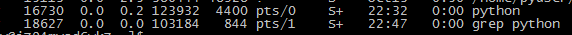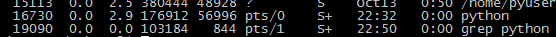# Python读取文件、大文件和指定行内容的几种方法

```f = open('/var/log/nginx/zhangnq.com.log','rb')
#读取全部
f.close()
# 按行读取
```

```import time
f = open('/var/log/nginx/zhangnq.com.log','rb')
while True:
time.sleep(1)
``````import time
f = open('/var/log/nginx/zhangnq.com.log','rb')
print(line)
time.sleep(1)
``````# 读取大文件
"""
Lazy function (generator) to read a file piece by piece.
Default chunk size: 1M
You can set your own chunk size
"""
file_object = open(file_path)
while True:
if not chunk_data:
break
yield chunk_data
```

with open

```with open(...) as f:
for line in f:
process(line) #
```

```f = open('/var/log/nginx/zhangnq.com.log','rb')
# 读取 第一行到第十行内容
print(data[0:10])
```

```# 读取文件多行间内容
content_list = list()
i = 1
for tmp in chunk.split('\n'):
if line_end != -1:
if i >= line_start and i <= line_end:                     content_list.append(tmp)             else:                 if i >= line_start:
content_list.append(tmp)
i += 1
return content_list
```

Comment (2)
1.夏日博客 　( 2019.10.18 19:16 ) : #-9

Python感觉比PHP还是难。

2.头条 　( 2019.10.29 00:01 ) : #-8

文章不错支持一下吧